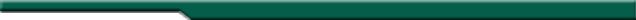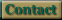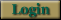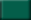#### Sandbagger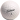What is one Descriptions of a sandbagger.Become a Member Sign up and start keeping your handicapHandicap Calculation How handicap is calculatedTell a Friend Tell your golfing buddies about the Sandbagger

#### SupportQuestions Sandbagger FAQ's.ContactSend us your questions and comments.

#### SocialSandbagger News Whats news with the SandbaggerGolf PicsWeekly golf course pics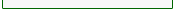# World HANDICAP FORMULA:A Handicap Index is a service mark used to indicate a measurement of a player's potential ability on a course of standard difficulty. Potential ability is measured by a player's best scores, and is expressed as a number taken to one decimal place. These scores are identified by calculating the handicap differential for each score. The Handicap Index is calculated by taking 96 percent of the average of the best handicap differentials, and applying rule 6.1 of the world handicap system.

How to Determine Handicap Differentials

A handicap differential is computed from four elements: adjusted gross score, Course Rating, Slope Rating and 113 (the Slope Rating of a course of standard difficulty). To determine the handicap differential, subtract the Course Rating from the adjusted gross score; multiply the difference by 113; then divide the resulting number by the Slope Rating. Round the final number to the nearest tenth.

Handicap Differential =
(Adjusted Gross Score - Course Rating) x 113 / Slope Rating

An adjusted gross score is determined by limiting the maximum score on a hole to net par plus
two strokes (�net double bogey�). This scoring limit is familiar to golfers playing a Stableford
format and now applies to all forms of play. If a player is allocated one stroke on a par 5 hole,
the maximum hole score is 5+1+2=8.

You can take no more than a net double-bogey, no matter your ability.

a. Plus Handicap Differential

When the adjusted gross score is higher than the Course Rating, the handicap differential is a positive number. Following is an example for determining a differential using an adjusted gross score of 95 made on a course with a Course Rating of 71.5 and a Slope Rating of 125:

 Adjusted Gross Score - Course Rating: 95 - 71.5 = 23.5 Difference x Standard Slope: 23.5 x 113 = 2655.5 Result / Slope Rating: 2655.5 / 125 = 21.24 Handicap Differential (rounded): 21.2

b. Minus Handicap Differential

When the adjusted gross score is lower than the Course Rating, the handicap differential is a negative number. Following is an example for determining a differential using an adjusted gross score of 69 made on a course with a Course Rating of 71.5 and a Slope Rating of 125:

 Adjusted Gross Score - Course Rating: 69 - 71.5 = -2.5 Difference x Standard Slope: -2.5 x 113 = -282.5 Result / Slope Rating: -282.5 / 125 = -2.26 Handicap Differential (rounded): -2.3

Handicap Index Formula

The Handicap Index Formula is based on the best handicap differentials in a player's scoring record. If a player's scoring record contains 20 or more scores, the best 8 handicap differentials of the most recent 20 scores are used to calculate the Handicap Index.

The procedure for calculating Handicap Indexes is as follows:

1. Use the table below to determine the number of handicap differentials to use;
Number of Score Differentials in scoring record Score Differential(s) to be used in calculation of Handicap Index Adjustment
3 Lowest 1 -2.0
4 Lowest 1 -1.0
5 Lowest 1 0
6 Average of lowest 2 -1.0
7 or 8 Average of lowest 2 0
9 to 11 Average of lowest 3 0
12 to 14 Average of lowest 4 0
15 or 16 Average of lowest 5 0
17 or 18 Average of lowest 6 0
19 Average of lowest 7 0
20 Average of lowest 8 0

2. Determine handicap differentials
3. Average the handicap differentials being used
4. Round off to the nearest tenth
 Total of lowest 8 handicap differentials: 108.2 Average (208.2 /8): 26.025 Round to nearest tenths: 26 Handicap Index: 26

Important Disclaimer: The Sandbagger Golf Handicap Calculator and Tracker is not prepared, approved, or licensed by the and is neither sponsored nor endorsed by WHA.